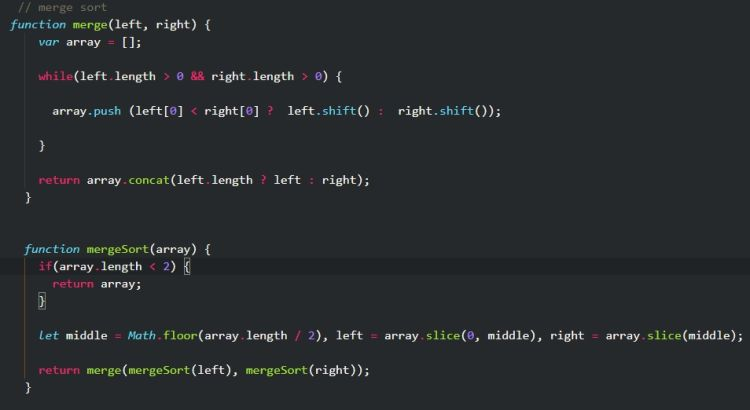← back to the blog

# Effective JavaScript Merge sort algorithm

##### Posted on September 27th, 2018 in JavaScript by George## About Merge sort in Javascript.

Merge sort it is very effective sorting algorithm with an Worst Time complexity of  O(n log(n)) being more effective in comparison with Quick sort   O(n^2) and insertion sort   O(n^2) for a hypothetical large data set.  Check out big O notation cheat sheet at www.bigocheatsheet.com .

This version example of Merge sort commes with comments explaining every step. Some of the syntax it is ES6.

## What does merge sort do ?

Merge sort splits recursively the given array into halfs until every element end up on it`s own.

As an example an array of [5,7,6,2,8,9,7,3];

It is split to [5,7,6,2] and [8,9,7,3] on the first call of mergeSort, then is calling for merge helper function. Recursively mergeSort is going to split the halfs respectively [5,7,6,2] and [8,9,7,3] to [5,6] and [6,2] for the first parameter and [8,9,7,3] and so on.

function merge(left, right) {
let array = [];
while(left.length > 0 && right.length > 0) {
//while the two arrays(left and right) have content
// remove (left.shfit()) first element of each arrays
// and send it to the empty array
//(array.push(ternary result))
array.push(left < right ? left.shift() : right.shift());
}
//return the array when while loop has finished
return array.concat(left.length ? left : right);
}
function mergeSort(array) {
if(array.length < 2)
return array;
//find the middle of the initial array
//then split it in two (left, and right)
let middle = Math.floor(array.length / 2),
left = array.slice(0, middle),
right = array.slice(middle);
// recursively call mergeSort
//inside the merge function
console.log(array);
return merge(mergeSort(left), mergeSort(right));
}
console.log(mergeSort([6,3,7,5,1,7]));